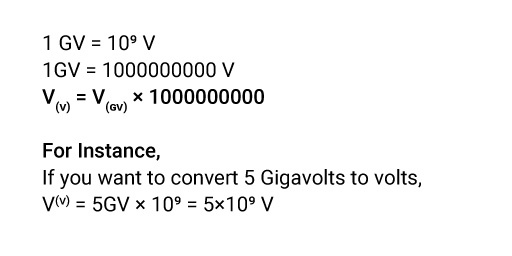## About Our GV to V Converter

“Zeroes during conversion: Put me in the right place, or I’ll spoil your calculation.”

We believe in smart, hard work in the world of hard work. Conversions are tricky, so there are great chances of making an error. So, here is our converter, which will save you time from doing small but essential calculations and coming up with an accurate answer.

You will find details on each measurement, from Gigavolts to volts conversion and all the other metric conversions.

### Definition of Gigavolts.

Gigavolt is a multiple unit of electromotive force or electrical potential difference. It is generated by a particle with one charged electron when it passes through the potential difference of one billion volts in a vacuum.

### What is the Symbol of Gigavolts?

GV is the symbol of Gigavolts.

### How to Convert Gigavolts to Volts?

To convert Gigavolts to volts, you must multiply the voltage by the conversion ratio. Since one Gigavolt equals one billion volts, multiply the Gigavolts by 1,000,000,000 to convert them to volts.

### The Gigavolts to Volts Conversion Formula.

You can apply the direct Gigavolts to Volts conversion formula to get an answer.It consists of many zeroes, so you may get confused using the formula. But the best thing is using our Gigavolts to Volts converter to get error-free outcomes.

### How to Use Our Gigavolts to Volts Converter?

Make your calculation smoother using our Gigavolts to volts calculator.

• Step2: Enter the value you want to convert.
• Step3: Press “Convert” to get the answer.

### How Many Gigavolts Are in a Volt?

1V = 10-9 GV

1V = 0.000000001 GV

One volt is equal to the one-billionth of a Gigavolt.

### What is the Difference Between Gigavolts and Volts?

• Gigavolt is a multiple unit of voltage. It is used to measure an enormous quantity of voltage. The abbreviation of Gigavolt is GV. Gigavolt consists of one billion volts.
• Volt is the basic unit of voltage. The abbreviation of Volts is V. One volt equals the one-billionth part of a Gigavolt.

### What is Gigavolt's Unit of Measure?

Gigavolts (GV) is the unit of electrical potential difference equal to 109 Volts.

### Examples of Converting Gigavolts to Volts.

Look at the examples given below,

V(V) = 3GV × 109 = 3×109 V

V(V) = 6GV × 109 = 6×109 V

V(V) = 7GV × 109 = 7×109 V

V(V) = 9GV × 109 = 9×109 V

### Gigavolts to Volts Conversion Table.

Have a quick view of the Gigavolts to Volts Conversion table.

Gigavolts Volts
1 GV 109 V
0.1 GV 108 V
0.01 GV 107 V
0.001 GV 106 V
0.0001 GV 105 V
0.00001 GV 104 V
0.000001 GV 103 V